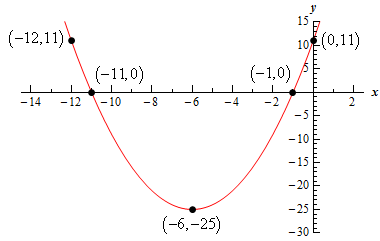Paul's Online Notes
Home / Algebra / Common Graphs / Parabolas
Show Mobile Notice Show All Notes Hide All Notes
Mobile Notice
You appear to be on a device with a "narrow" screen width (i.e. you are probably on a mobile phone). Due to the nature of the mathematics on this site it is best views in landscape mode. If your device is not in landscape mode many of the equations will run off the side of your device (should be able to scroll to see them) and some of the menu items will be cut off due to the narrow screen width.

### Section 4-2 : Parabolas

4. Sketch the graph of the following parabola. The graph should contain the vertex, the $$y$$‑intercept, $$x$$-intercepts (if any) and at least one point on either side of the vertex.

$f\left( x \right) = {x^2} + 12x + 11$

Show All Steps Hide All Steps

Start Solution

Let’s find the vertex first. In this case the equation is in the form $$f\left( x \right) = a{x^2} + bx + c$$. And so we know the vertex is the point $$\left( { - \frac{b}{{2a}},f\left( { - \frac{b}{{2a}}} \right)} \right)$$. The vertex is then,

$\left( { - \frac{{12}}{{2\left( 1 \right)}},f\left( { - \frac{{12}}{{2\left( 1 \right)}}} \right)} \right) = \left( { - 6,f\left( { - 6} \right)} \right) = \left( { - 6, - 25} \right)$

Also note that $$a = 1 > 0$$ for this parabola and so the parabola will open upwards.

Show Step 2

The $$y$$-intercept is just the point $$\left( {0,f\left( 0 \right)} \right)$$. A quick function evaluation gives us that $$f\left( 0 \right) = 11$$ and so for our equation the $$y$$-intercept is $$\left( {0,11} \right)$$.

Show Step 3

For the $$x$$-intercepts we just need to solve the equation $$f\left( x \right) = 0$$. So, let’s solve that for our equation.

\begin{align*}{x^2} + 12x + 11 & = 0\\ \left( {x + 1} \right)\left( {x + 11} \right) & = 0\hspace{0.25in} \to \hspace{0.25in}x = - 1,\,\,\,x = - 11\end{align*}

The two $$x$$-intercepts for this parabola are then : $$\left( { - 1,0} \right)$$ and $$\left( { - 11,0} \right)$$.

Show Step 4

Because we had two $$x$$-intercepts for this parabola we already have at least one point on either side of the vertex and so we don’t really need to find any more points for our graph.

However, just for the practice let’s find the point corresponding to the $$y$$-intercept on the other side the vertex.

The $$y$$-intercept is a distance of 6 to the right of the vertex and so there will be a corresponding point at the same $$y$$ value to the left and it will be a distance of 6 to the left of the vertex. Therefore, the point to the left of the vertex corresponding to the $$y$$-intercept is $$\left( { - 12,11} \right)$$.

Show Step 5

Here is a sketch of the parabola including all the points we found above.